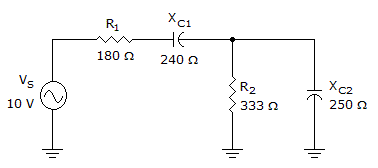# Electronics - RC Circuits

Exercise : RC Circuits - True or False
1.
A 10 kcapacitive reactance equals a 100 µS capacitive susceptance.
True
False
Explanation:
No answer description is available. Let's discuss.

2.
In a purely capacitive ac circuit, the amount of energy converted to heat is proportional to the circuit's current.
True
False
Explanation:
No answer description is available. Let's discuss.

3.
Resistance is the same as impedance in an RC circuit.
True
False
Explanation:
No answer description is available. Let's discuss.

4.The magnitude of the impedance in the given circuit is approximately 500.

True
False